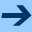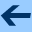Functions

# Functions

All operations in GOO are functions.

Functions accept zero or more arguments, and return one value. The parameter list of the function describes the number and types of the arguments that the function accepts, and the type of the value it returns.

There are two kinds of functions, methods and generic functions. Both are invoked in the same way. The caller does not need to know whether the function it is calling is a method or a generic function.

A method is the basic unit of executable code. A method accepts a number of arguments, creates local bindings for them, executes an implicit body in the scope of these bindings, and then returns a value.

A generic function contains a number of methods. When a generic function is called, it compares the arguments it received with the parameter lists of the methods it contains. It selects the most appropriate method and invokes it on the arguments. This technique of method dispatch is the basic mechanism of polymorphism in GOO.

All GOO functions are objects, instances of <fun>. Generic functions are instances of <gen> and methods are instances of <met>.
 () C fun-name (x| => (t? )) P returns the name of function or false if unavailable. fun-names (x| => ) P returns the names of parameters of x or () if unavailable. fun-specs (x| => ) P returns the specializers of x. fun-nary? (x| => ) P returns true iff the function takes optional arguments. fun-arity (x| => ) P returns x's number of required arguments. fun-val (x| => ) P returns the return type of x. FUN (FUN ,sig ,@body) S creates an anonymous method with signature ,sig and when called evaluates ,@body as (SEQ ,@body) (cf. Scheme's LAMBDA). The following a few example functions and their application: ```((fun (x) x) 1) ==> 1 ((fun (x| => ) x) 2) ==> 2 ((fun (x|...) x) 1 2 3) ==> (1 2 3) ((fun (x y|...) y) 1 2 3) ==> (2 3) ((fun (x => (tup ))) (tup x)) 1) \$ == \$ (tup 1) ```

where
 ,sig == (,@params) | (,@params => ,ret) L ,params == (,@vars [(,name '...')]) L where parameter lists can include an optional nary parameter which binds to all arguments beyond required arguments. ,ret == ,var | (TUP ,@ret-vars) L with TUP turning into corresponding t* function return type. ,ret-var == (,name ,type) L
 LOC (LOC ((,name ,sig ,@fbody)) ,@body) S == ``` (LET ((,name #f) ...) (SET ,name (fun ,sig ,@fbody)) ... ,@body) ``` LOC introduces local functions that can recursively call each other (cf. Scheme's LETREC). DF (DF ,name ,sig ,@body) S == (DV ,name (FUN ,sig ,@body)) followed by setting the function's name. zipped (x| => ) G == (fun (y|...) (app x y)). This is useful when mapping over zipped multiple sequences (e.g., (map (zipped +) (zip '(1 2) '(2 3))). OP (OP ,op-arg ...) S creates an anonymous function with implicitly defined arguments, where ,op-arg is either an implicit required parameter "_" or rest parameter "..." or an s-expression potentially containing further op-args. The required parameters are found ordered according to a depth-first walk of the op-args. The following are typical examples: ```((op _) 1) ==> 1 ((op 2) 1) ==> 2 ((op + _ 1) 3) ==> 4 ((op lst ... 1) 3 2) ==> (3 2 1) ((op tail (tail _)) '(1 2 3)) ==> (3) ``` app (f| args|... => ) G calls f with arguments (cat (sub args 0 (- (len args) 2)) (elt args (- (len args) 1))).

• Generics
• Methods
• Macros

•Functions